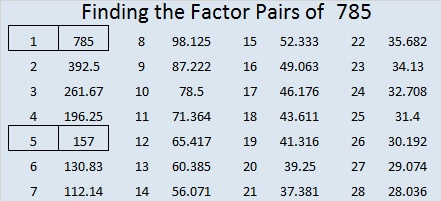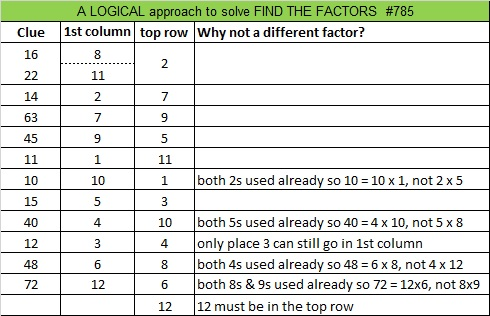# 785 and Level 4

• 785 is a composite number.
• Prime factorization: 785 = 5 x 157
• The exponents in the prime factorization are 1 and 1. Adding one to each and multiplying we get (1 + 1)(1 + 1) = 2 x 2 = 4. Therefore 785 has exactly 4 factors.
• Factors of 785: 1, 5, 157, 785
• Factor pairs: 785 = 1 x 785 or 5 x 157
• 785 has no square factors that allow its square root to be simplified. √785 ≈ 28.01785.Now for today’s puzzle:Print the puzzles or type the solution on this excel file: 12-factors-782-787

—————————————–

Here’s a little more about the number 785:

785 is the sum of two squares two different ways:

• 28² + 1² = 785
• 23² + 16² = 785

785 is also the sum of three squares three different ways:

• 26² + 10² + 3² = 785
• 25² + 12² + 4² = 785
• 19² + 18² + 10² = 785

Because its prime factorization is 5 × 157 (two numbers that are also the sum of two squares), 785 is the hypotenuse of four Pythagorean triples, two of which are primitive triples:

• 56-783-785 primitive calculated from 2(28)(1), 28² – 1², 28² + 1²
• 273-736-785 primitive calculated from 23² – 16², 2(23)(16), 23² + 16²
• 425-660-785 which is 5 times 85-132-157
• 471-628-785 which is 157 times 3-4-5

785 is also a palindrome in two different bases:

• 555 BASE 12; note that 5(144) + 5(12) + 5(1) = 785
• 101 BASE 28; note that 1(28²) + 0(28) + 1(1) = 785

—————————————–